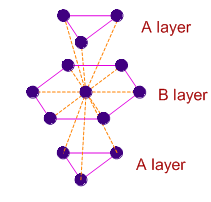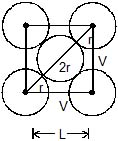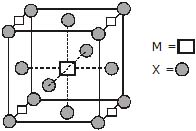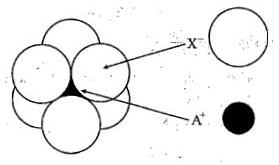Courses

# MCQ (Previous Year Questions) -Solid State (Level 2)

## 10 Questions MCQ Test Chemistry for JEE Advanced | MCQ (Previous Year Questions) -Solid State (Level 2)

Description
This mock test of MCQ (Previous Year Questions) -Solid State (Level 2) for JEE helps you for every JEE entrance exam. This contains 10 Multiple Choice Questions for JEE MCQ (Previous Year Questions) -Solid State (Level 2) (mcq) to study with solutions a complete question bank. The solved questions answers in this MCQ (Previous Year Questions) -Solid State (Level 2) quiz give you a good mix of easy questions and tough questions. JEE students definitely take this MCQ (Previous Year Questions) -Solid State (Level 2) exercise for a better result in the exam. You can find other MCQ (Previous Year Questions) -Solid State (Level 2) extra questions, long questions & short questions for JEE on EduRev as well by searching above.
QUESTION: 1

### In hexagonal systems of crystals, a frequently encountered arrangement of atoms is described as a hexagonal prism. Here, the top and bottom of the cell are regular hexagons and three atoms are sandwiched in between them. A space-filling model of this structure, called hexagonal close-packed (HCP), is constituted of a sphere on a flat surface surrounded in the same plane by six identical spheres as closely as possible. Three spheres are then placed over the first layer so that they touch each other and represent the second layer. Each one of these three spheres touches three spheres of the bottom layer. Finally, the second layer is covered with a third layer that is identical to the bottom layer in relative position. Assume radius of every sphere to be 'r'. The number of atoms in this HCP unit cells is                                                      [JEE 2008]

Solution:
1/6×12 + 1/2×2 + 3 =6
12 corner atoms, 2 face center atoms, 3middle atoms.
QUESTION: 2

### In hexagonal systems of crystals, a frequently encountered arrangement of atoms is described as a hexagonal prism. Here, the top and bottom of the cell are regular hexagons and three atoms are sandwiched in between them. A space-filling model of this structure, called hexagonal close-packed (HCP), is constituted of a sphere on a flat surface surrounded in the same plane by six identical spheres as closely as possible. Three spheres are then placed over the first layer so that they touch each other and represent the second layer. Each one of these three spheres touches three spheres of the bottom layer. Finally, the second layer is covered with a third layer that is identical to the bottom layer in relative position. Assume radius of every sphere to be 'r'.  The volume of this HCP unit cell is                                                                                [JEE 2008]

Solution:
Height of unit cell = 4r√2/3
Base area = 6 x √3/4 (2r)2
Volume = height x base area
=24√2r^3
QUESTION: 3

### In hexagonal systems of crystals, a frequently encountered arrangement of atoms is described as a hexagonal prism. Here, the top and bottom of the cell are regular hexagons and three atoms are sandwiched in between them. A space-filling model of this structure, called hexagonal close-packed (HCP), is constituted of a sphere on a flat surface surrounded in the same plane by six identical spheres as closely as possible. Three spheres are then placed over the first layer so that they touch each other and represent the second layer. Each one of these three spheres touches three spheres of the bottom layer. Finally, the second layer is covered with a third layer that is identical to the bottom layer in relative position. Assume radius of every sphere to be 'r'. The empty space in this HCP unit cell is                                                                                         [JEE 2008]

Solution:
Packing fraction = 74%
Empty space = 26%
QUESTION: 4

Which of the following FCC structure contains cations in alternate tetrahedral voids ?                                  [JEE 2005]

Solution: In ZnS, Anions (S^−2) are placed in fcc manner and cations (Zn^+2) are placed in alternate tetrahedral voids.
QUESTION: 5

A substance AxBy crystallises in a FCC lattice in which atoms "A" occupy each corner of the cube and atoms "B" occupy the centres of each face of the cube. Identify the correct composition of the substance AxBy.                                                                              [JEE - 2002]

Solution:
QUESTION: 6

In a solid "AB" having NaCl structure "A" atoms occupy the corners of the cubic unit cell. If all the face-centred atoms along one of the axes are removed, then the resultant stoichiometry of the solid is                            [JEE - 2000]

Solution:
QUESTION: 7

The coordination number of a metal crystallising in a hcp structure is                                            [JEE - 2000]

Solution:
In three dimensional hexagonal close packing of crystals, the two dimensional hcp layers are arranged in ABAB... pattern as shown below. The atoms in A layers are exactly above (or below) one type of the trigonal voids in B layer.

The central atom in B layer is immediately surrounded by 12 atoms i.e., 6 in layer B and 3 each in two A layers.QUESTION: 8

The packing efficiency of the -dimensional square unit cell shown below is                            [JEE - 2010]Solution:
QUESTION: 9

A compound MpXq has cubic close packing (ccp) arrangement of X. Its unit cell structure is shown below. The empirical formula of the compound is [JEE - 2012]Solution: Since the given is ccp then x will ocuupy all corners ,edge centres,face centres and m will occupy edge cente, body centre and x value is 6 and m value 3 finally we get mx2.
QUESTION: 10

The arrangement of X_ ioins around A+ ion in solid AX is given in the figure (not drawn to scale). If the radius of X_is 250 pm, the radius of A+ isSolution: